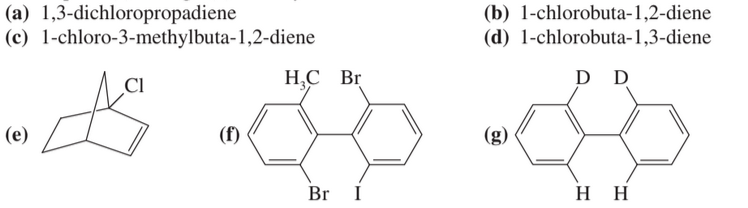×
Get Full Access to Organic Chemistry - 8 Edition - Chapter 5 - Problem 15p
Get Full Access to Organic Chemistry - 8 Edition - Chapter 5 - Problem 15p

×

# Draw three-dimensional representations of the followingISBN: 9780321768414 33

## Solution for problem 15P Chapter 5

Organic Chemistry | 8th Edition

• Textbook Solutions
• 2901 Step-by-step solutions solved by professors and subject experts
• Get 24/7 help from StudySoup virtual teaching assistantsOrganic Chemistry | 8th Edition

4 5 1 401 Reviews
25
0
Problem 15P

Draw three-dimensional representations of the following compounds. Which have asymmetric carbon atoms? Which have no asymmetric carbons but are chiral anyway? Use your mod-

els for parts (a) through (d) and any others that seem unclear.Equation Transcription:Text Transcription:

Cl

H_3C Br

Br

Step-by-Step Solution:

Solution:

Step 1There is no asymmetric C atoms in this given molecule but it has chiral C atom.

Three dimensional picture of  1,3-dichloropropadiene is given below,In this 3D structure it has been found that, there is no plane of symmetry because all the molecules are present in different planes.

Step 2 of 7

Step 3 of 7

## Discover and learn what students are asking

Calculus: Early Transcendental Functions : Iterated Integrals and Area in the Plane
?In Exercises 1 - 10, evaluate the integral. $$\int_{0}^{\sqrt{4-x^{2}}} x^{2} y d y$$

Statistics: Informed Decisions Using Data : Testing the Significance of the Least-Squares Regression Model
?The U.S. Population The following data represent the population of the United States for the years 1900–2010 An ecologist i

Unlock Textbook Solution